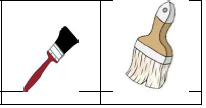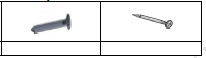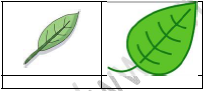# CBSE Class 1 Maths Measurement Thicker Thinner Worksheet Set C

Download printable Mathematics Class 1 Worksheets in pdf format, CBSE Class 1 Maths Measurement Thicker Thinner Worksheet Set C has been prepared as per the latest syllabus and exam pattern issued by CBSE, NCERT and KVS. Also download free pdf Mathematics Class 1 Assignments and practice them daily to get better marks in tests and exams for Grade 1. Free chapter wise worksheets with answers have been designed by Standard 1 teachers as per latest examination pattern

## Measurement Thicker Thinner Class 1 Mathematics Worksheet Pdf

Class 1 Mathematics students should refer to the following printable worksheet in Pdf for Measurement Thicker Thinner in Grade 1. This test paper with questions and solutions for Standard 1 Mathematics will be very useful for tests and exams and help you to score better marks

### Class 1 Mathematics Worksheet for Measurement Thicker Thinner

Practice Worksheet
CBSE Class 1 Mathematics
Topic: Measurement

Q1) Which Brush is thinner?Ans: A

Q2) Which object is thicker?Ans: A

Q3) Which Tree is thinner?Ans: A

Q4) Which snake is thinner?Ans: A

Q5) Which object is thicker?Ans: A

Click below to download practice worksheet for CBSE Class 1 Maths Measurement Thicker Thinner Worksheet Set C

## Tags:

Click for more Mathematics Study Material
 CBSE Class 1 Maths Addition Worksheet CBSE Class 1 Maths Number from One to Nine Worksheet Set B CBSE Class 1 Maths Pattern Worksheet Set D CBSE Class 1 Maths Addition Worksheet Set B CBSE Class 1 Maths Shapes and Space Worksheet CBSE Class 1 Maths Number from Twenty one to fifty Worksheet Set A CBSE Class 1 Maths Subtraction Worksheet Set B CBSE Class 1 Maths Measurement Heavier Lighter Worksheet Set D CBSE Class 1 Maths Numbers Worksheet Set H CBSE Class 1 Maths Numbers from Twentyone to Fifty Worksheet CBSE Class 1 Maths Number from Ten to Twenty Worksheet Set E CBSE Class 1 Maths How Many Worksheet Set C CBSE Class 1 Maths Numbers Worksheet Set B CBSE Class 1 Maths Shapes and Space Worksheet Set A CBSE Class 1 Maths Data Handling Worksheet CBSE Class 1 Maths Number from One to Nine Worksheet Set C CBSE Class 1 Maths Addition Worksheet Set C CBSE Class 1 Maths Subtraction Worksheet CBSE Class 1 Maths Number from Twenty one to fifty Worksheet Set B CBSE Class 1 Maths Subtraction Worksheet Set C CBSE Class 1 Maths Money Worksheet Set A CBSE Class 1 Maths Numbers Worksheet Set I CBSE Class 1 Maths Numbers Worksheet CBSE Class 1 Maths Number from Ten to Twenty Worksheet Set F CBSE Class 1 Maths How Many Worksheet Set D CBSE Class 1 Maths Numbers Worksheet Set C CBSE Class 1 Maths Shapes and Space Worksheet Set B CBSE Class 1 Maths How Many Worksheet CBSE Class 1 Maths Number from One to Nine Worksheet Set D CBSE Class 1 Maths Addition Worksheet Set D CBSE Class 1 Maths Time Worksheet CBSE Class 1 Maths Number from Twenty one to fifty Worksheet Set C CBSE Class 1 Maths Subtraction Worksheet Set D CBSE Class 1 Maths Money Worksheet Set B CBSE Class 1 Maths Numbers Worksheet Set J CBSE Class 1 Maths Patterns Worksheet CBSE Class 1 Maths Number from Ten to Twenty Worksheet Set G CBSE Class 1 Maths How Many Worksheet Set E CBSE Class 1 Maths Numbers Worksheet Set D CBSE Class 1 Maths Measurement Worksheet CBSE Class 1 Maths Number from Ten to Twenty Worksheet Set A CBSE Class 1 Maths Data Handling Worksheet Set A CBSE Class 1 Maths Number from Twenty one to fifty Worksheet Set D CBSE Class 1 Maths Time Worksheet Set A CBSE Class 1 Maths Money Worksheet Set C CBSE Class 1 Maths Pattern Worksheet Set A CBSE Class 1 Maths Revision Worksheet Set B CBSE Class 1 Maths Number from Ten to Twenty Worksheet Set H CBSE Class 1 Maths Measurement Longer Shorter Worksheet Set A CBSE Class 1 Maths Numbers Worksheet Set E CBSE Class 1 Maths Money Worksheet CBSE Class 1 Maths Number from Ten to Twenty Worksheet Set B CBSE Class 1 Maths Data Handling Worksheet Set B CBSE Class 1 Maths Number from Twenty one to fifty Worksheet Set E CBSE Class 1 Maths Time Worksheet Set B CBSE Class 1 Maths Money Worksheet Set D CBSE Class 1 Maths Pattern Worksheet Set B CBSE Class 1 Maths Revision Worksheet Set C CBSE Class 1 Maths Number from Ten to Twenty Worksheet Set I CBSE Class 1 Maths Shapes and Space Worksheet Set C CBSE Class 1 Maths Measurement Longer Shorter Worksheet Set B CBSE Class 1 Maths Numbers Worksheet Set F CBSE Class 1 Maths Numbers from One to Nine Worksheet CBSE Class 1 Maths Number from Ten to Twenty Worksheet Set C CBSE Class 1 Maths How Many Worksheet Set A CBSE Class 1 Maths Number from Twenty one to fifty Worksheet Set F CBSE Class 1 Maths Time Worksheet Set C CBSE Class 1 Maths Number from One to Nine Worksheet Set A CBSE Class 1 Maths Pattern Worksheet Set C CBSE Class 1 Maths Addition Worksheet Set A CBSE Class 1 Maths Revision Worksheet CBSE Class 1 Maths Number from Ten to Twenty Worksheet Set J CBSE Class 1 Maths Subtraction Worksheet Set A CBSE Class 1 Maths Measurement Thicker Thinner Worksheet Set C CBSE Class 1 Maths Numbers Worksheet Set G CBSE Class 1 Maths Numbers from Ten to Twenty Worksheet CBSE Class 1 Maths Number from Ten to Twenty Worksheet Set D CBSE Class 1 Maths How Many Worksheet Set B CBSE Class 1 Maths Numbers Worksheet Set A

## Latest NCERT & CBSE News

Read the latest news and announcements from NCERT and CBSE below. Important updates relating to your studies which will help you to keep yourself updated with latest happenings in school level education. Keep yourself updated with all latest news and also read articles from teachers which will help you to improve your studies, increase motivation level and promote faster learning

### Board Exams Date Sheet Class 10 and Class 12

Datesheet for CBSE Board Exams Class 10  (Scroll down for Class 12 Datesheet) Datesheet for CBSE Board Exams Class 12

### CBSE Term 2 Board Examinations

CBSE vide Circular No.Acad-51/2021 dated 5th July, 2021, notified that in the session 2021-2022, Board Examinations would be conducted in two terms, i.e.. Term I and Term II. This decision was taken due to the uncertainty arising out of COVID 19 Pandemic. Term I...

### Class 10th and 12th Term 2 Revaluation Process 2022

Evaluation of the Answer Books is done under a well-settled Policy. To ensure that the evaluation is error free, CBSE is taking several steps. After strictly following these steps, the result is prepared. Though, CBSE is having a well-settled system of assessment,...

### Celebration of Matribhasha Diwas Mother Language day

UNESCO has declared 21st February of every year to be celebrated as International Mother Language day to promote dissemination of Mother Language of all, create awareness of linguistic and cultural traditions and diversity across the world and to inspire solidarity...